# RD Sharma Solutions For Class 7 Maths Exercise 20.4 Chapter 20 Mensuration - I (Perimeter and area of rectilinear figures)

In exercise 20.4 of Chapter 20 of RD Sharma Solutions for Class 7 Maths we shall study about the area of the triangle. Students can refer to RD Sharma Solutions for Class 7 which is designed by our expert tutors for the purpose of strengthening the concepts and problem-solving abilities. On regular practice, students can speed up the method of solving problems by using short-cut tips to secure high marks in their examination.

## Download the PDF of RD Sharma Solutions For Class 7 Chapter 20 – Mensuration – I (Perimeter and Area of rectilinear figures) Exercise 20.4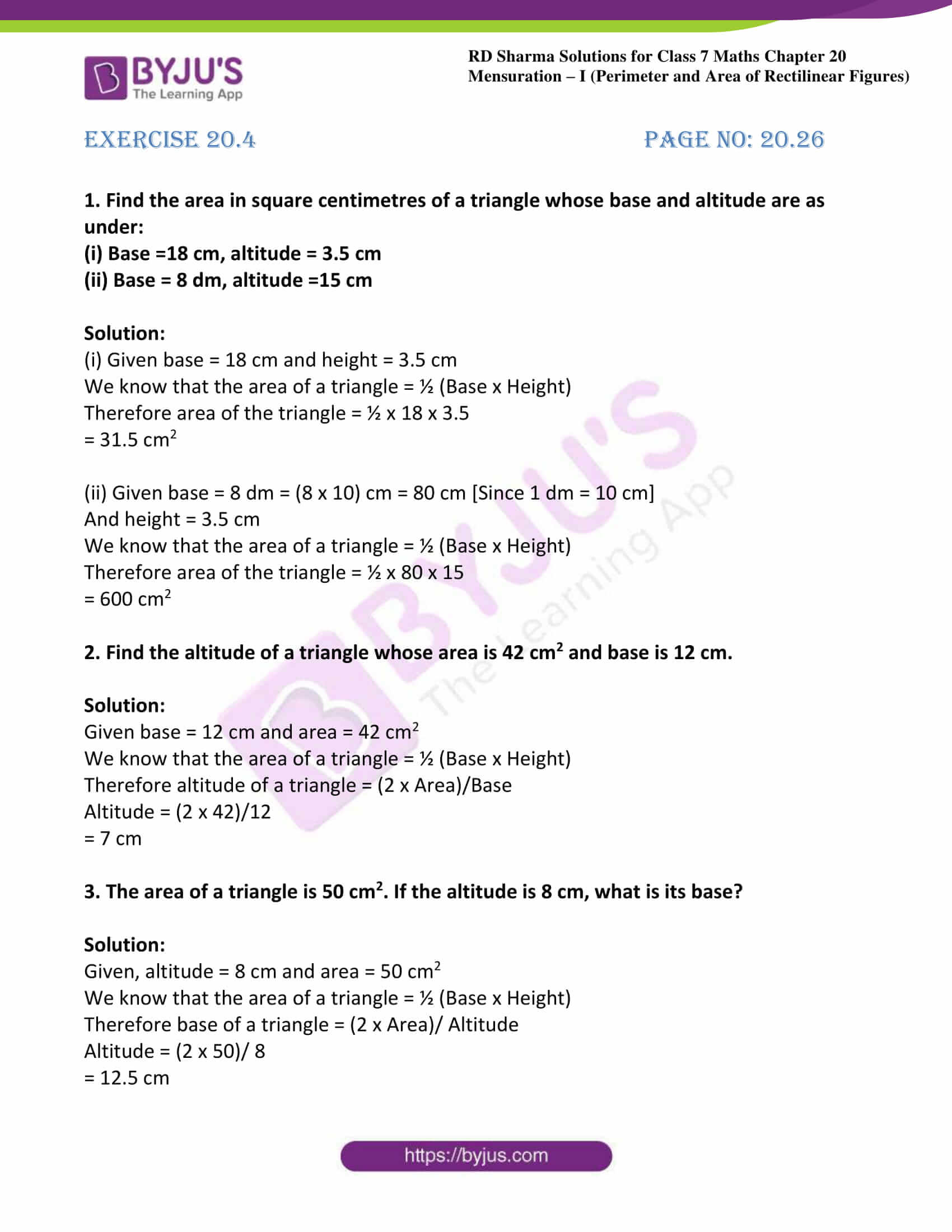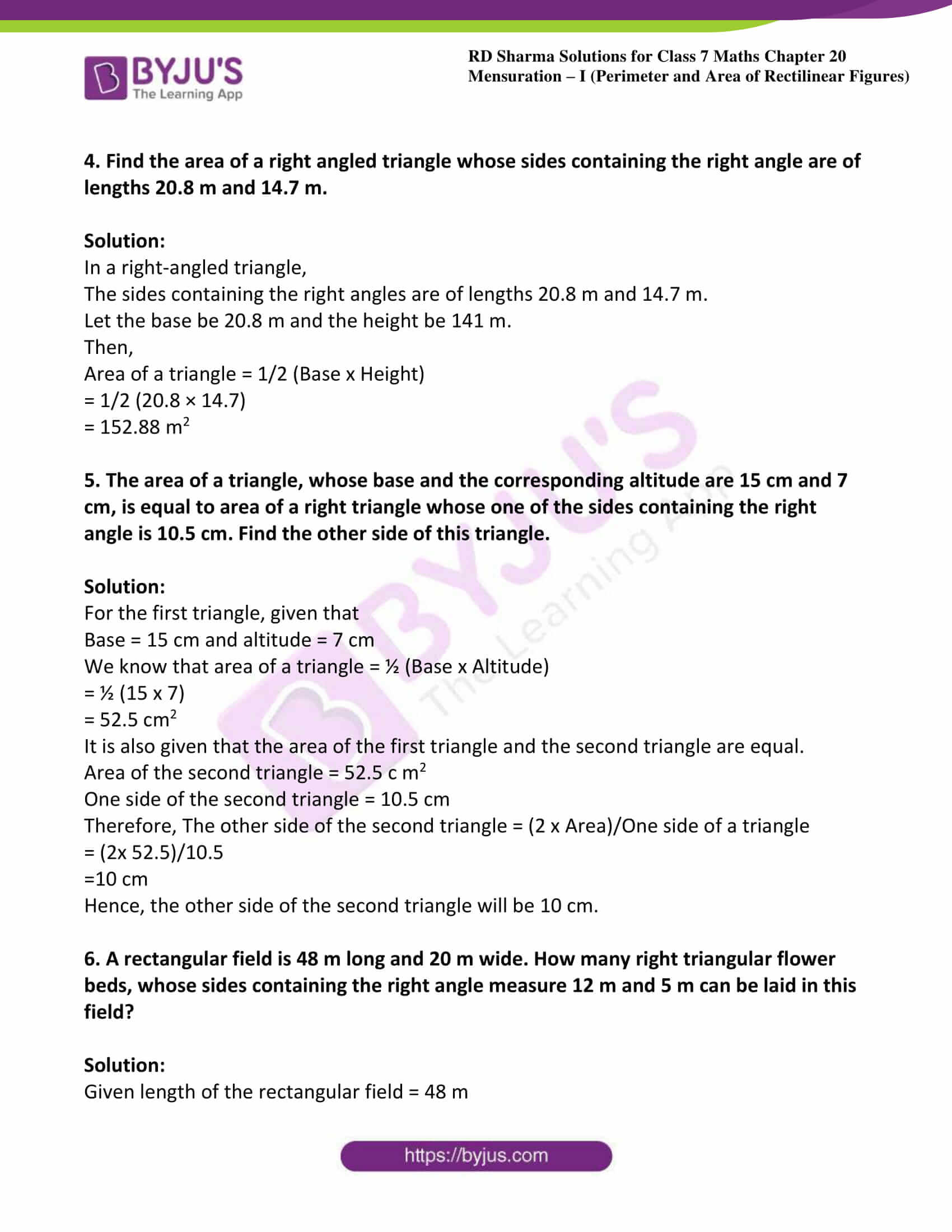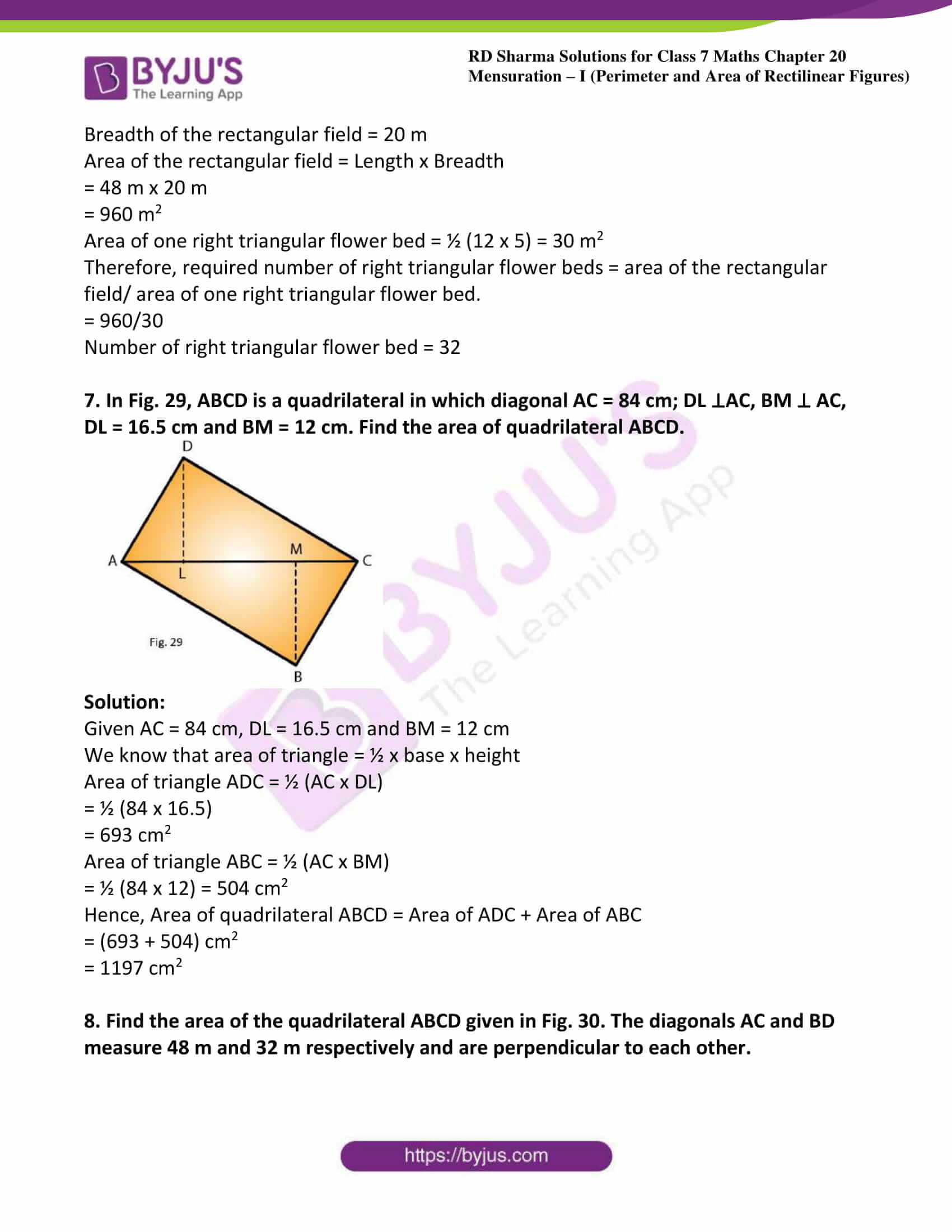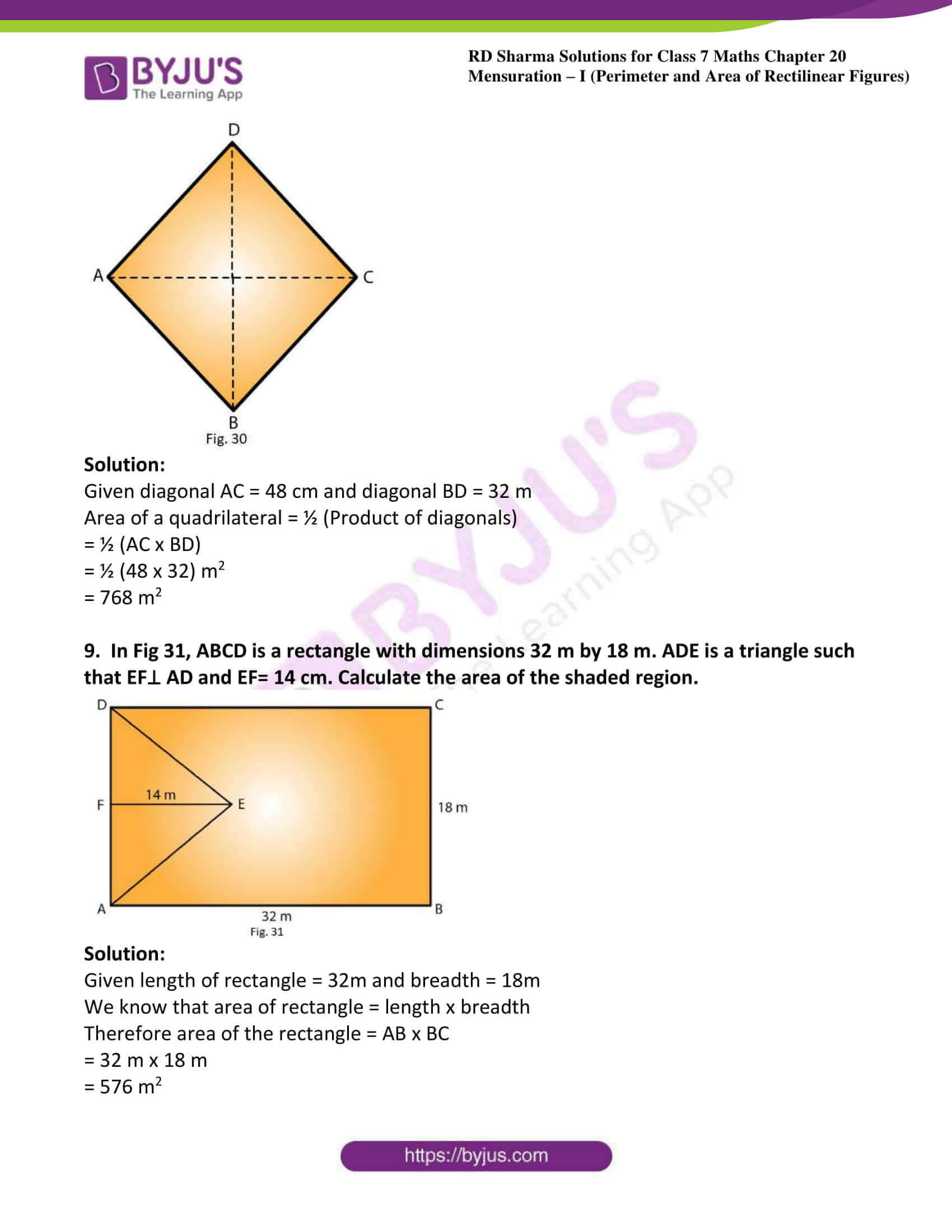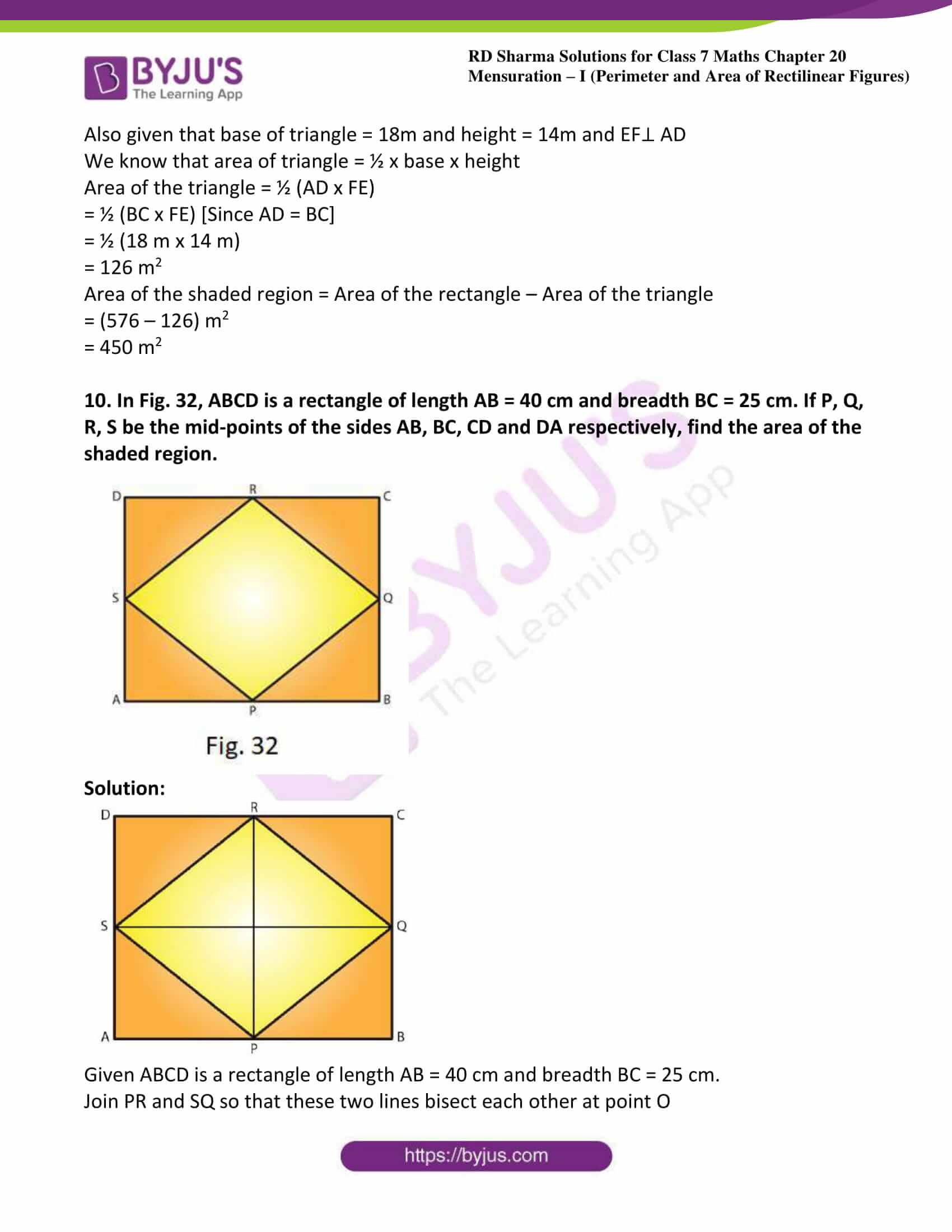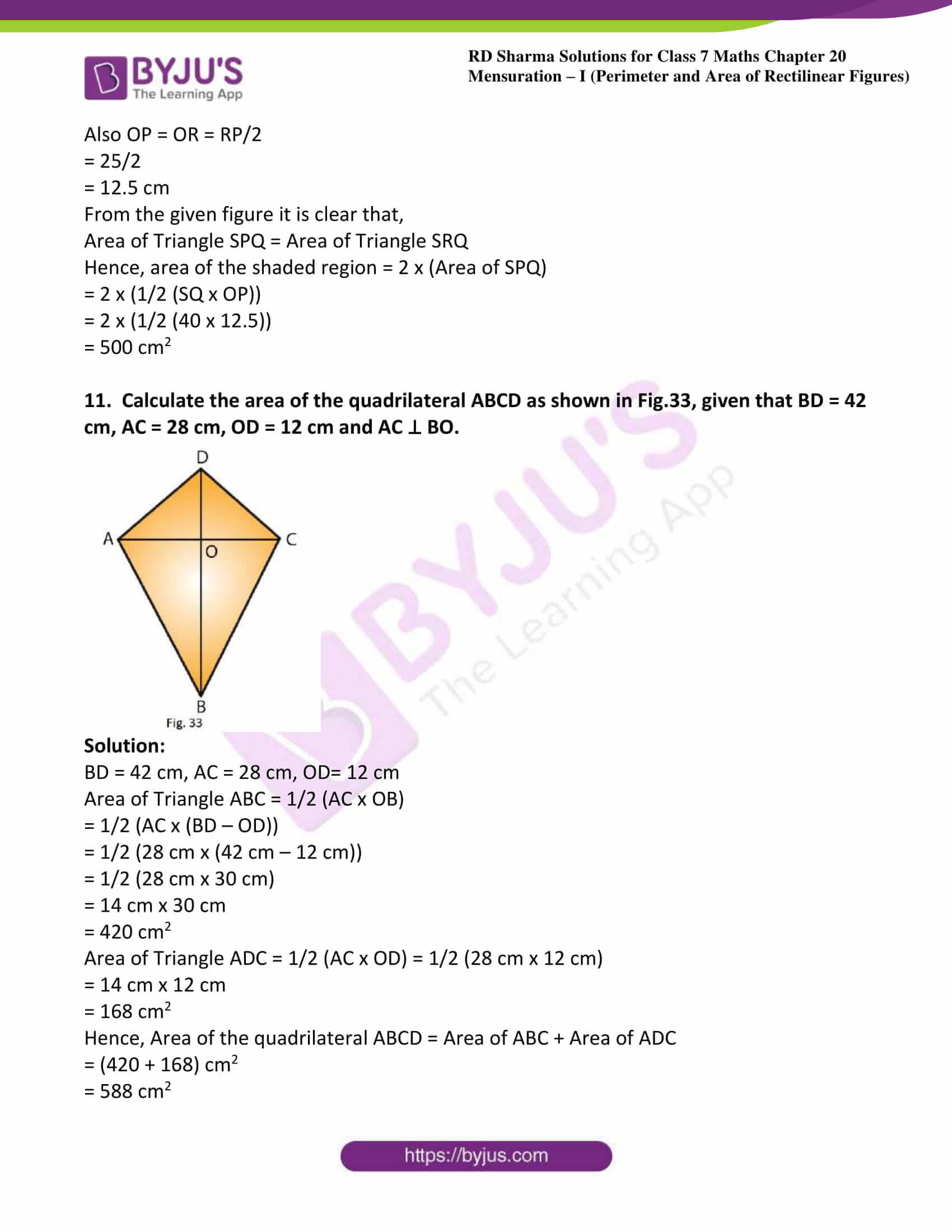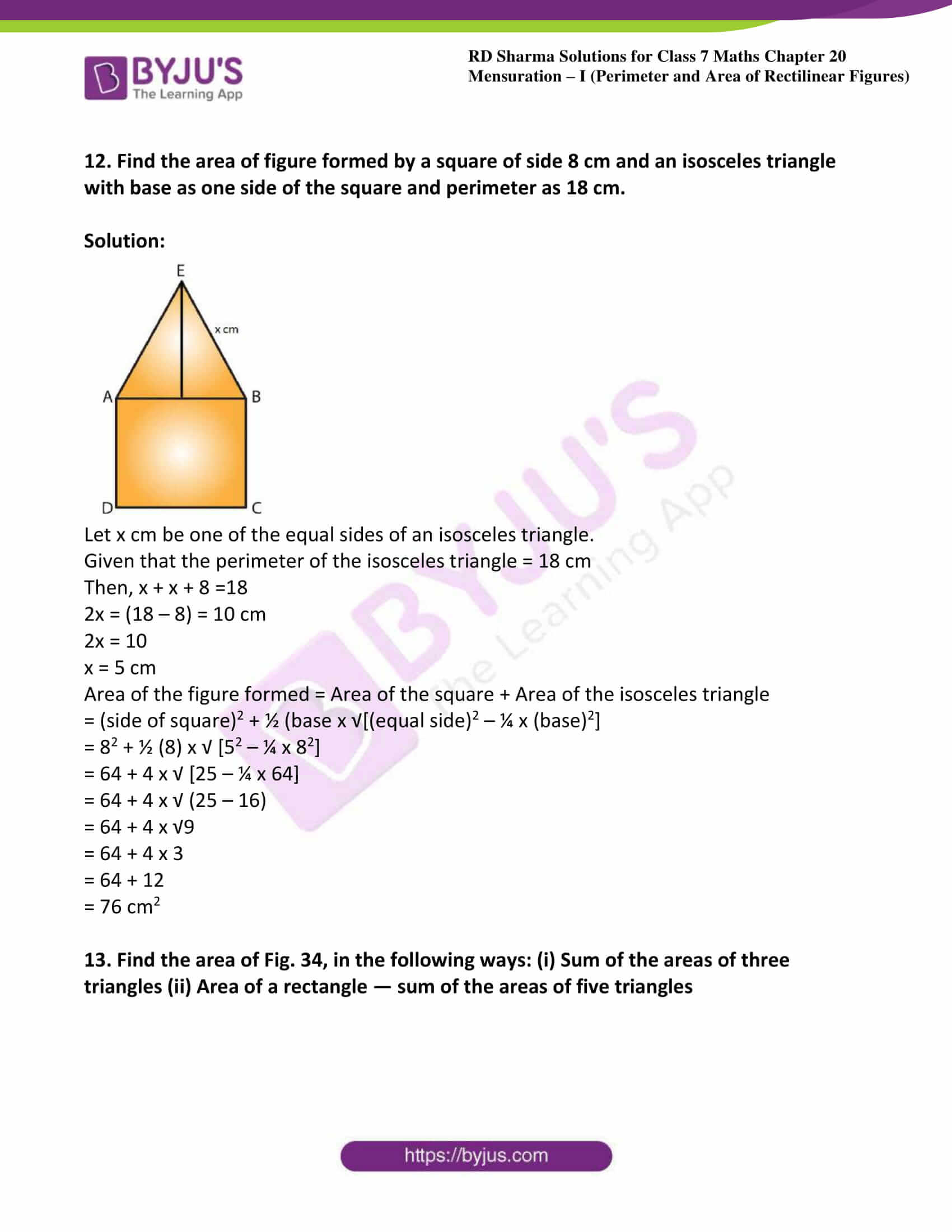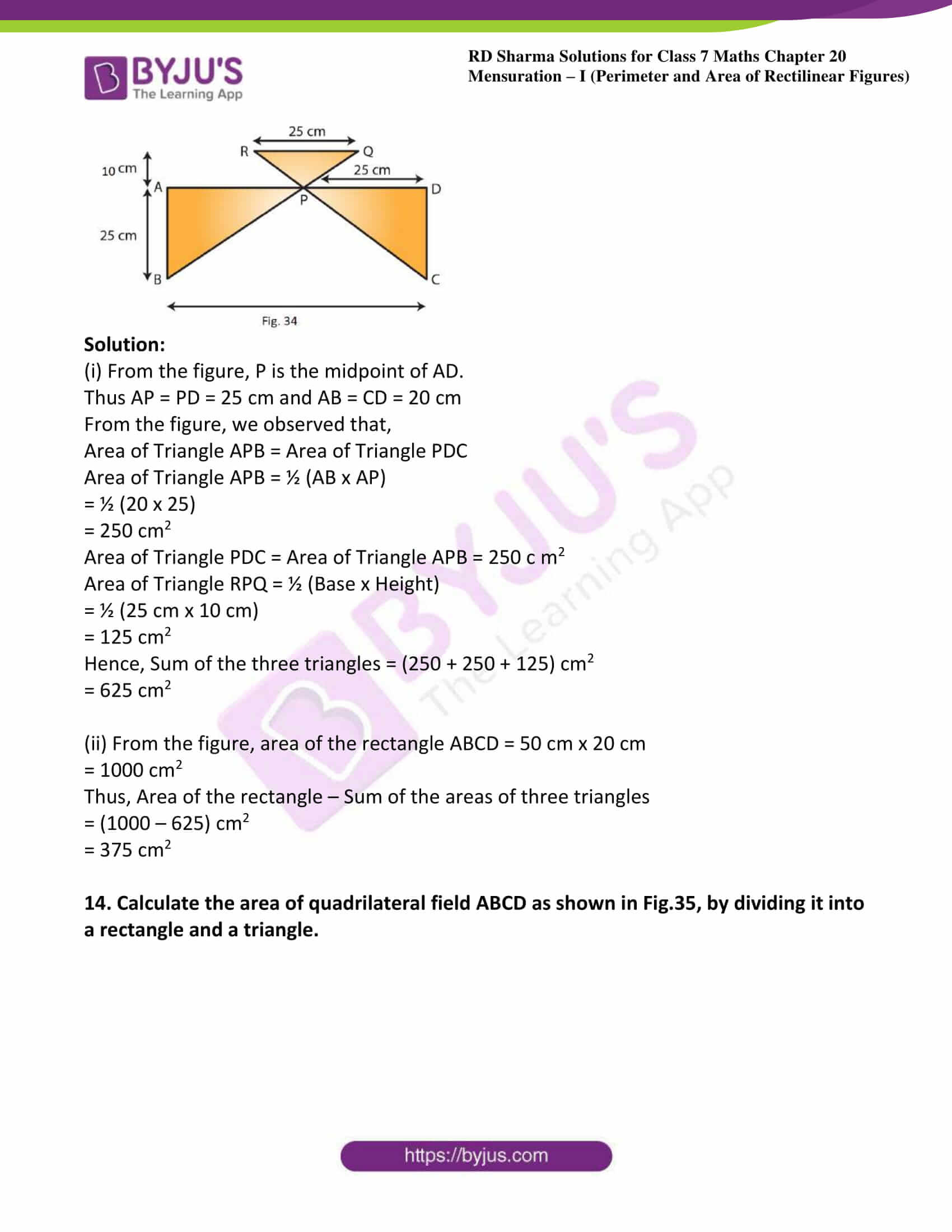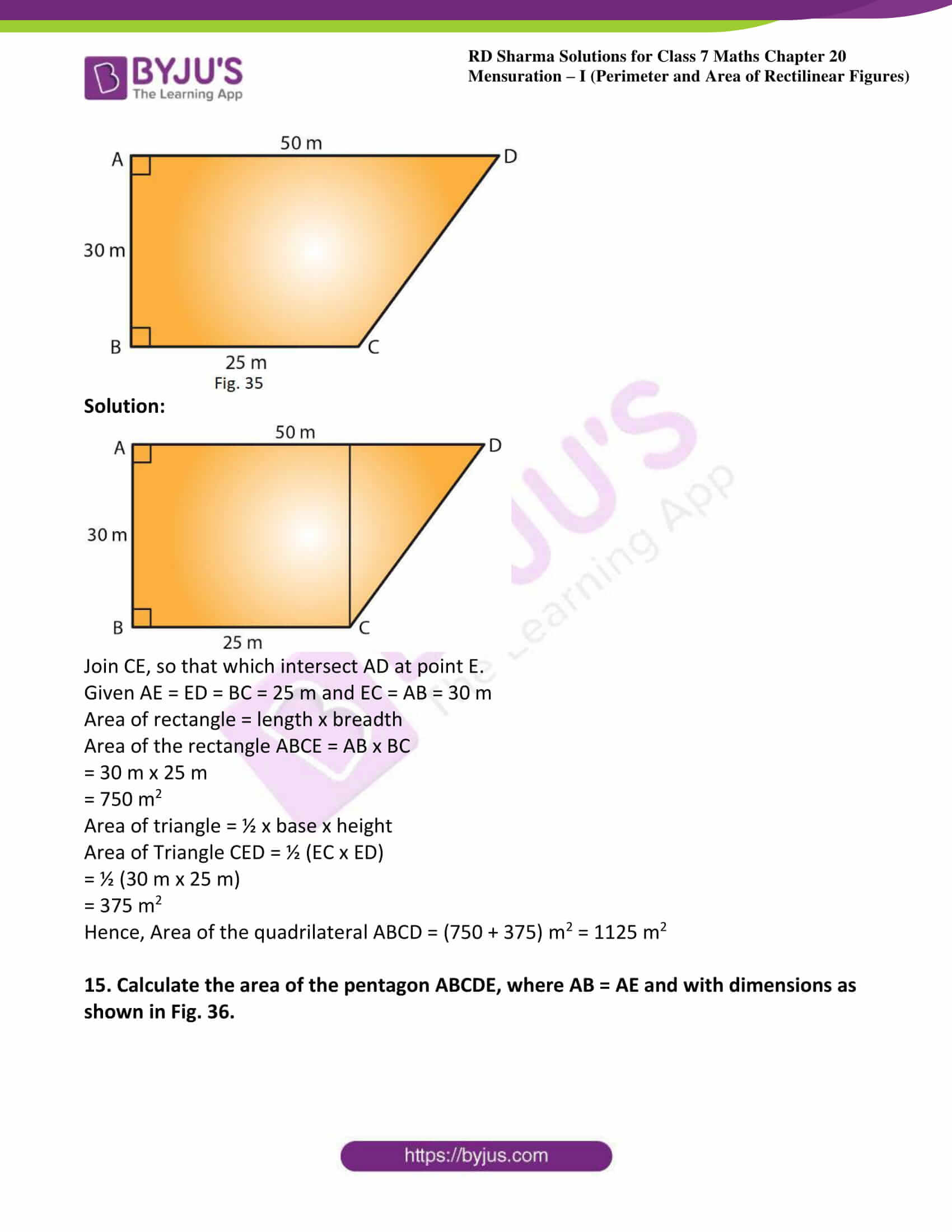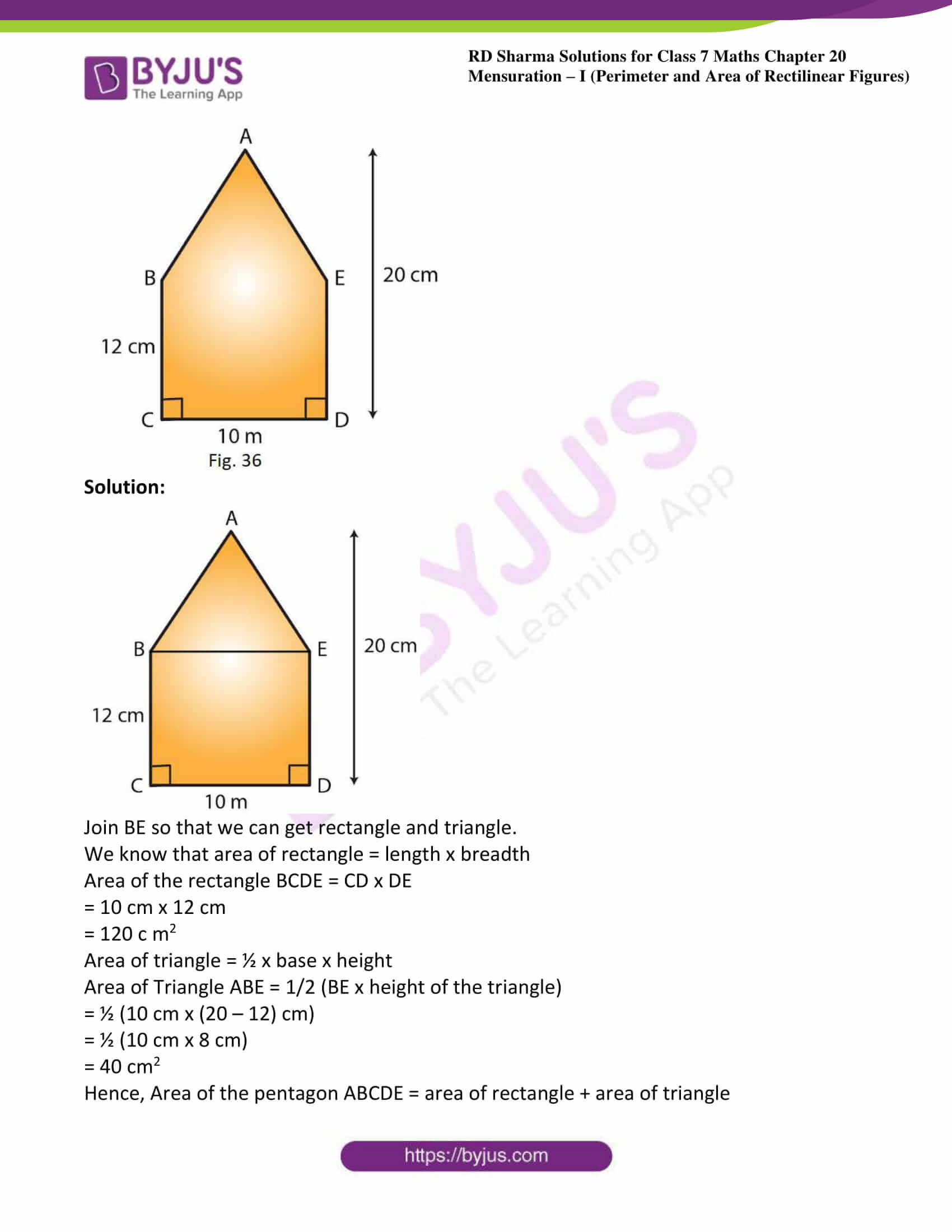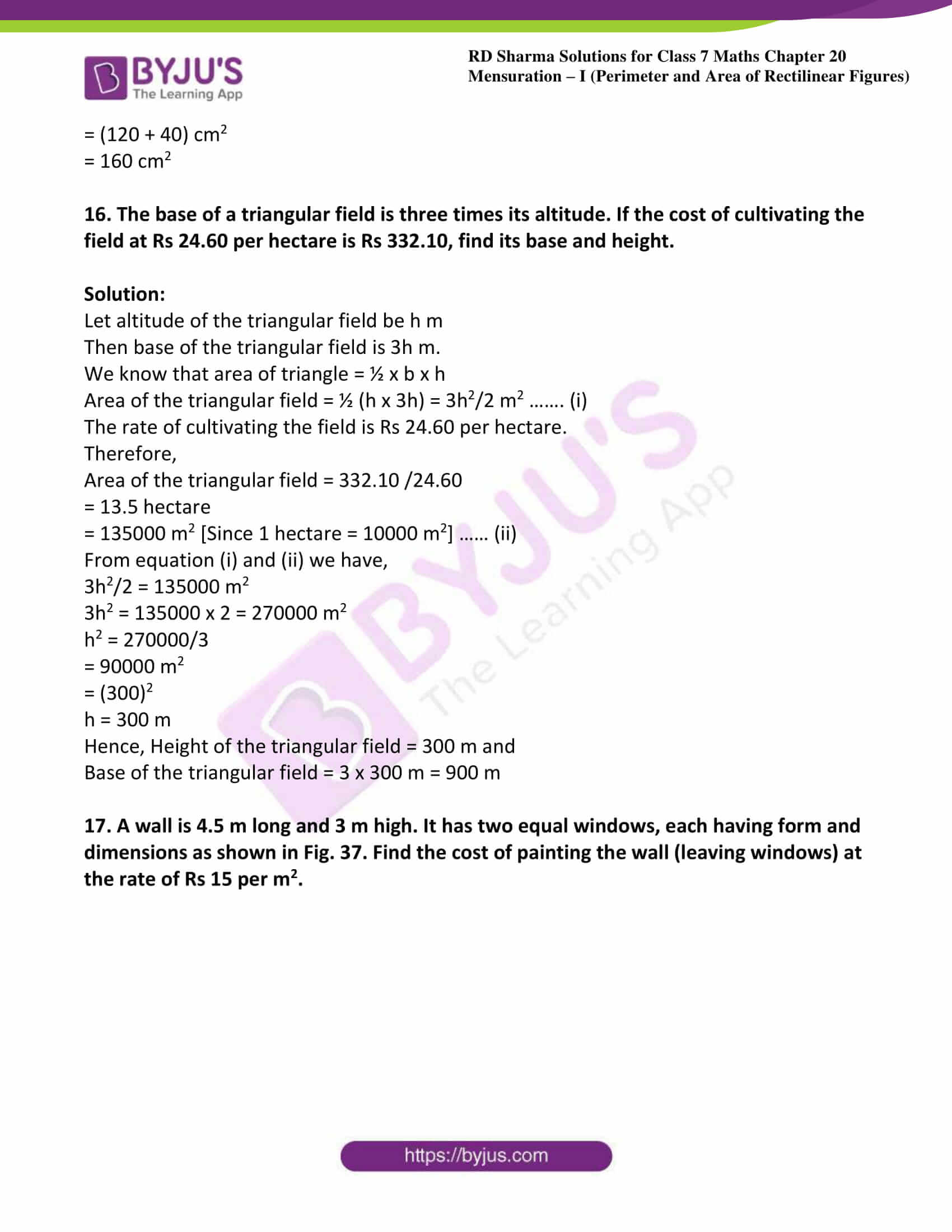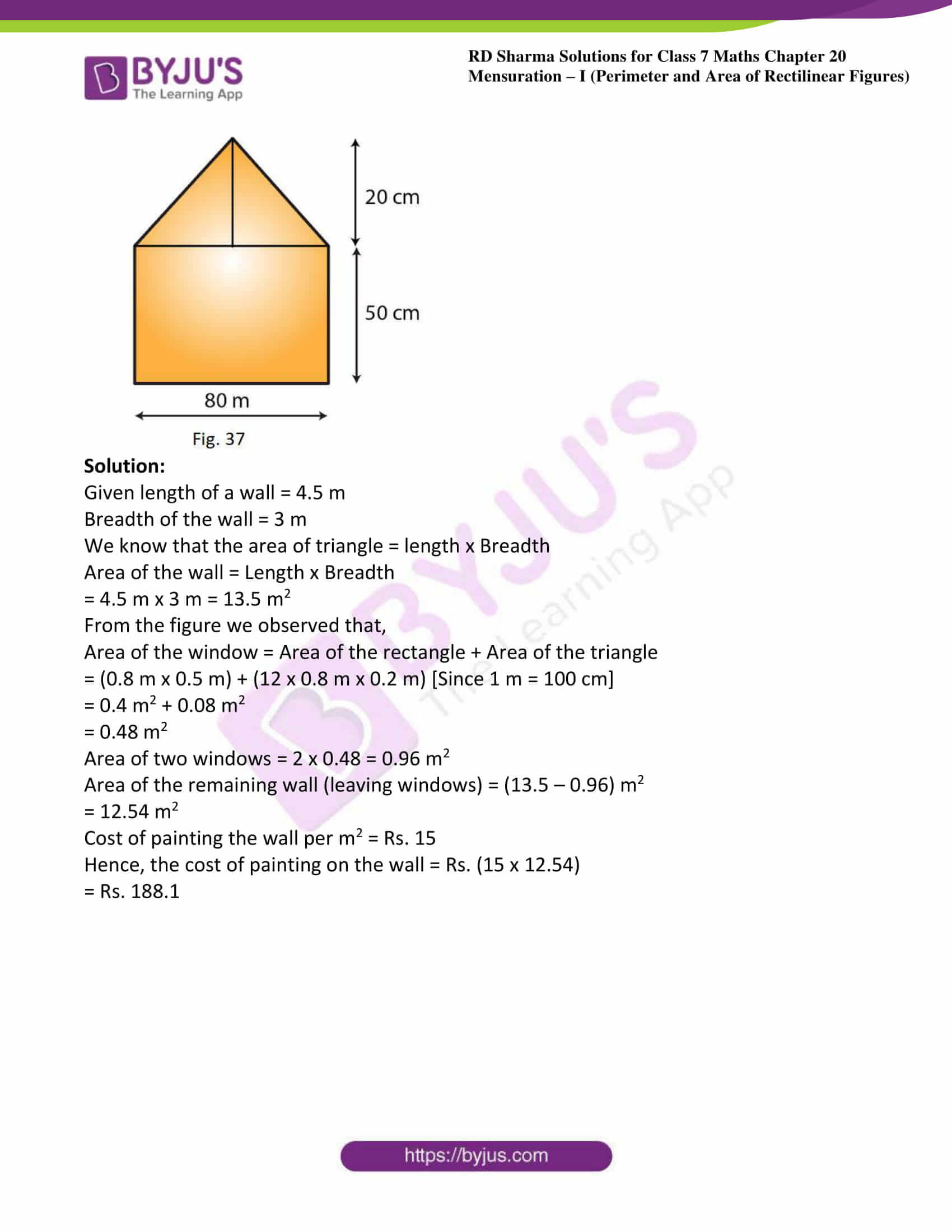### Access answers to Maths RD Sharma Solutions For Class 7 Chapter 20 – Mensuration – I (Perimeter and Area of rectilinear figures) Exercise 20.4

1. Find the area in square centimetres of a triangle whose base and altitude are as under:

(i) Base =18 cm, altitude = 3.5 cm

(ii) Base = 8 dm, altitude =15 cm

Solution:

(i) Given base = 18 cm and height = 3.5 cm

We know that the area of a triangle = ½ (Base x Height)

Therefore area of the triangle = ½ x 18 x 3.5

= 31.5 cm2

(ii) Given base = 8 dm = (8 x 10) cm = 80 cm [Since 1 dm = 10 cm]

And height = 3.5 cm

We know that the area of a triangle = ½ (Base x Height)

Therefore area of the triangle = ½ x 80 x 15

= 600 cm2

2. Find the altitude of a triangle whose area is 42 cm2 and base is 12 cm.

Solution:

Given base = 12 cm and area = 42 cm2

We know that the area of a triangle = ½ (Base x Height)

Therefore altitude of a triangle = (2 x Area)/Base

Altitude = (2 x 42)/12

= 7 cm

3. The area of a triangle is 50 cm2. If the altitude is 8 cm, what is its base?

Solution:

Given, altitude = 8 cm and area = 50 cm2

We know that the area of a triangle = ½ (Base x Height)

Therefore base of a triangle = (2 x Area)/ Altitude

Altitude = (2 x 50)/ 8

= 12.5 cm

4. Find the area of a right angled triangle whose sides containing the right angle are of lengths 20.8 m and 14.7 m.

Solution:

In a right-angled triangle,

The sides containing the right angles are of lengths 20.8 m and 14.7 m.

Let the base be 20.8 m and the height be 141 m.

Then,

Area of a triangle = 1/2 (Base x Height)

= 1/2 (20.8 × 14.7)

= 152.88 m2

5. The area of a triangle, whose base and the corresponding altitude are 15 cm and 7 cm, is equal to area of a right triangle whose one of the sides containing the right angle is 10.5 cm. Find the other side of this triangle.

Solution:

For the first triangle, given that

Base = 15 cm and altitude = 7 cm

We know that area of a triangle = ½ (Base x Altitude)

= ½ (15 x 7)

= 52.5 cm2

It is also given that the area of the first triangle and the second triangle are equal.

Area of the second triangle = 52.5 c m2

One side of the second triangle = 10.5 cm

Therefore, The other side of the second triangle = (2 x Area)/One side of a triangle

= (2x 52.5)/10.5

=10 cm

Hence, the other side of the second triangle will be 10 cm.

6. A rectangular field is 48 m long and 20 m wide. How many right triangular flower beds, whose sides containing the right angle measure 12 m and 5 m can be laid in this field?

Solution:

Given length of the rectangular field = 48 m

Breadth of the rectangular field = 20 m

Area of the rectangular field = Length x Breadth

= 48 m x 20 m

= 960 m2

Area of one right triangular flower bed = ½ (12 x 5) = 30 m2

Therefore, required number of right triangular flower beds = area of the rectangular field/ area of one right triangular flower bed.

= 960/30

Number of right triangular flower bed = 32

7. In Fig. 29, ABCD is a quadrilateral in which diagonal AC = 84 cm; DL ⊥AC, BM ⊥ AC, DL = 16.5 cm and BM = 12 cm. Find the area of quadrilateral ABCD.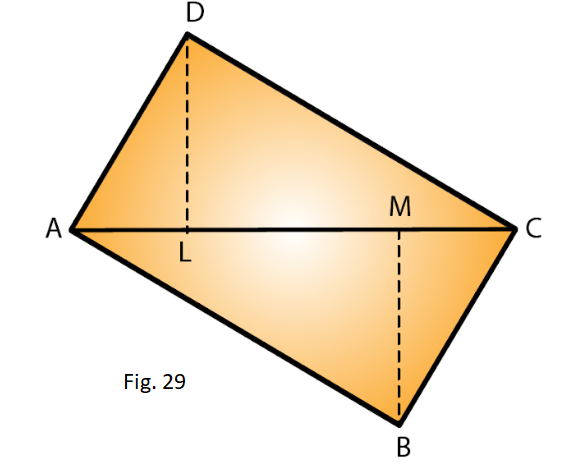Solution:

Given AC = 84 cm, DL = 16.5 cm and BM = 12 cm

We know that area of triangle = ½ x base x height

Area of triangle ADC = ½ (AC x DL)

= ½ (84 x 16.5)

= 693 cm2

Area of triangle ABC = ½ (AC x BM)

= ½ (84 x 12) = 504 cm2

Hence, Area of quadrilateral ABCD = Area of ADC + Area of ABC

= (693 + 504) cm2

= 1197 cm2

8. Find the area of the quadrilateral ABCD given in Fig. 30. The diagonals AC and BD measure 48 m and 32 m respectively and are perpendicular to each other.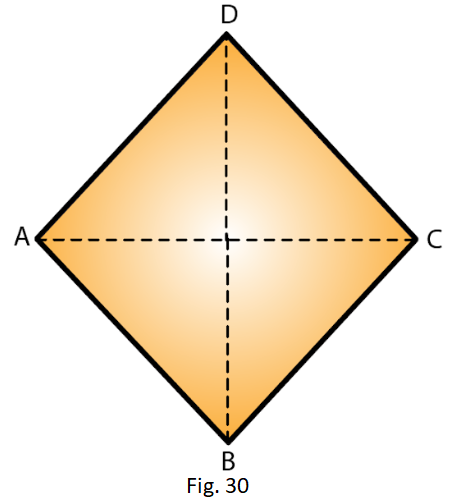Solution:

Given diagonal AC = 48 cm and diagonal BD = 32 m

Area of a quadrilateral = ½ (Product of diagonals)

= ½ (AC x BD)

= ½ (48 x 32) m2

= 768 m2

9.  In Fig 31, ABCD is a rectangle with dimensions 32 m by 18 m. ADE is a triangle such that EF⊥ AD and EF= 14 cm. Calculate the area of the shaded region.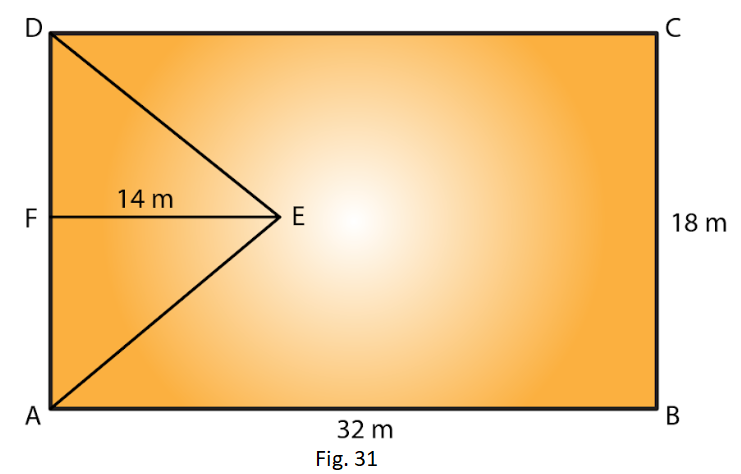Solution:

Given length of rectangle = 32m and breadth = 18m

We know that area of rectangle = length x breadth

Therefore area of the rectangle = AB x BC

= 32 m x 18 m

= 576 m2

Also given that base of triangle = 18m and height = 14m and EF⊥ AD

We know that area of triangle = ½ x base x height

Area of the triangle = ½ (AD x FE)

= ½ (BC x FE) [Since AD = BC]

= ½ (18 m x 14 m)

= 126 m2

Area of the shaded region = Area of the rectangle – Area of the triangle

= (576 – 126) m2

= 450 m2

10. In Fig. 32, ABCD is a rectangle of length AB = 40 cm and breadth BC = 25 cm. If P, Q, R, S be the mid-points of the sides AB, BC, CD and DA respectively, find the area of the shaded region.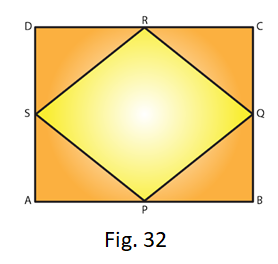Solution: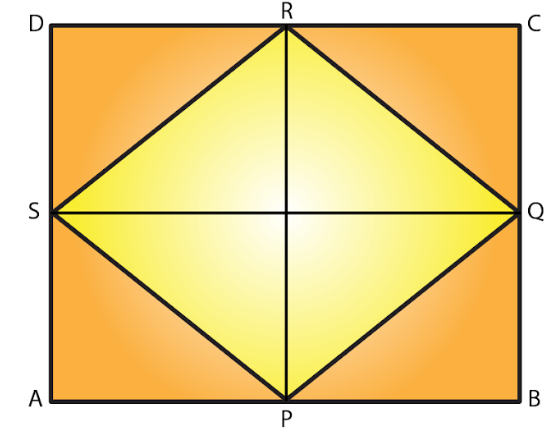Given ABCD is a rectangle of length AB = 40 cm and breadth BC = 25 cm.

Join PR and SQ so that these two lines bisect each other at point O

Also OP = OR = RP/2

= 25/2

= 12.5 cm

From the given figure it is clear that,

Area of Triangle SPQ = Area of Triangle SRQ

Hence, area of the shaded region = 2 x (Area of SPQ)

= 2 x (1/2 (SQ x OP))

= 2 x (1/2 (40 x 12.5))

= 500 cm2

11.  Calculate the area of the quadrilateral ABCD as shown in Fig.33, given that BD = 42 cm, AC = 28 cm, OD = 12 cm and AC ⊥ BO.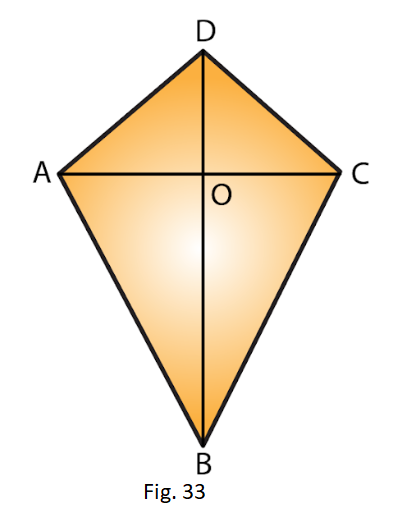Solution:

BD = 42 cm, AC = 28 cm, OD= 12 cm

Area of Triangle ABC = 1/2 (AC x OB)

= 1/2 (AC x (BD – OD))

= 1/2 (28 cm x (42 cm – 12 cm))

= 1/2 (28 cm x 30 cm)

= 14 cm x 30 cm

= 420 cm2

Area of Triangle ADC = 1/2 (AC x OD) = 1/2 (28 cm x 12 cm)

= 14 cm x 12 cm

= 168 cm2

Hence, Area of the quadrilateral ABCD = Area of ABC + Area of ADC

= (420 + 168) cm2

= 588 cm2

12. Find the area of figure formed by a square of side 8 cm and an isosceles triangle with base as one side of the square and perimeter as 18 cm.

Solution: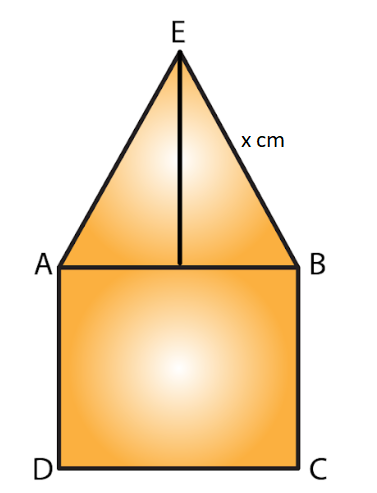Let x cm be one of the equal sides of an isosceles triangle.

Given that the perimeter of the isosceles triangle = 18 cm

Then, x + x + 8 =18

2x = (18 – 8) = 10 cm

2x = 10

x = 5 cm

Area of the figure formed = Area of the square + Area of the isosceles triangle

= (side of square)2 + ½ (base x √[(equal side)2 – ¼ x (base)2]

= 82 + ½ (8) x √ [52 – ¼ x 82]

= 64 + 4 x √ [25 – ¼ x 64]

= 64 + 4 x √ (25 – 16)

= 64 + 4 x √9

= 64 + 4 x 3

= 64 + 12

= 76 cm2

13. Find the area of Fig. 34, in the following ways: (i) Sum of the areas of three triangles (ii) Area of a rectangle — sum of the areas of five triangles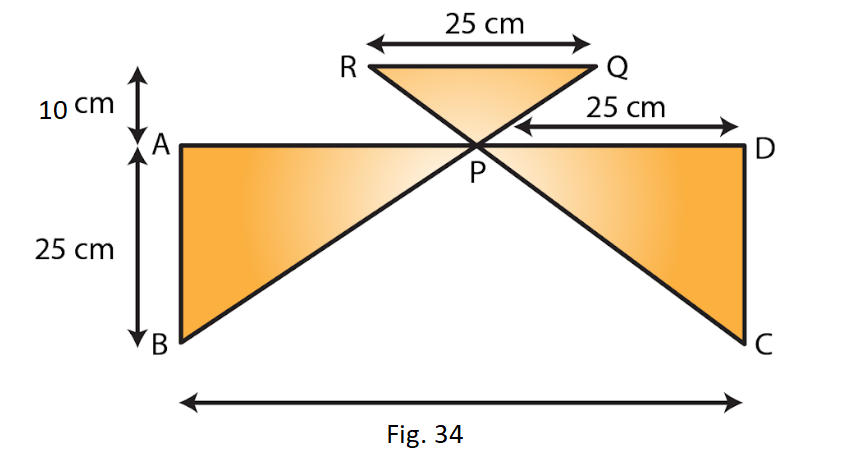Solution:

(i) From the figure, P is the midpoint of AD.

Thus AP = PD = 25 cm and AB = CD = 20 cm

From the figure, we observed that,

Area of Triangle APB = Area of Triangle PDC

Area of Triangle APB = ½ (AB x AP)

= ½ (20 x 25)

= 250 cm2

Area of Triangle PDC = Area of Triangle APB = 250 c m2

Area of Triangle RPQ = ½ (Base x Height)

= ½ (25 cm x 10 cm)

= 125 cm2

Hence, Sum of the three triangles = (250 + 250 + 125) cm2

= 625 cm2

(ii) From the figure, area of the rectangle ABCD = 50 cm x 20 cm

= 1000 cm2

Thus, Area of the rectangle – Sum of the areas of three triangles

= (1000 – 625) cm2

= 375 cm2

14. Calculate the area of quadrilateral field ABCD as shown in Fig.35, by dividing it into a rectangle and a triangle.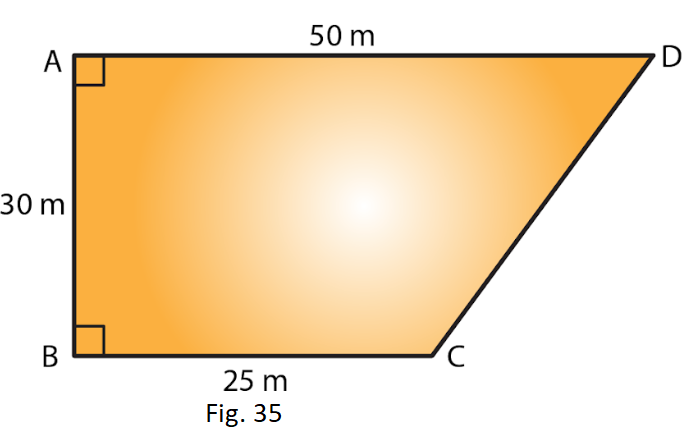Solution: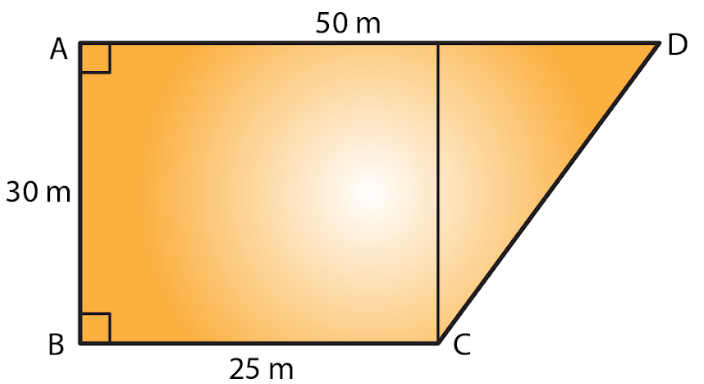Join CE, so that which intersect AD at point E.

Given AE = ED = BC = 25 m and EC = AB = 30 m

Area of rectangle = length x breadth

Area of the rectangle ABCE = AB x BC

= 30 m x 25 m

= 750 m2

Area of triangle = ½ x base x height

Area of Triangle CED = ½ (EC x ED)

= ½ (30 m x 25 m)

= 375 m2

Hence, Area of the quadrilateral ABCD = (750 + 375) m2 = 1125 m2

15. Calculate the area of the pentagon ABCDE, where AB = AE and with dimensions as shown in Fig. 36.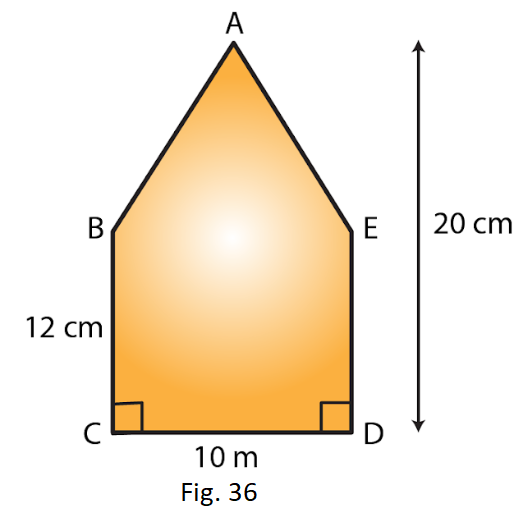Solution: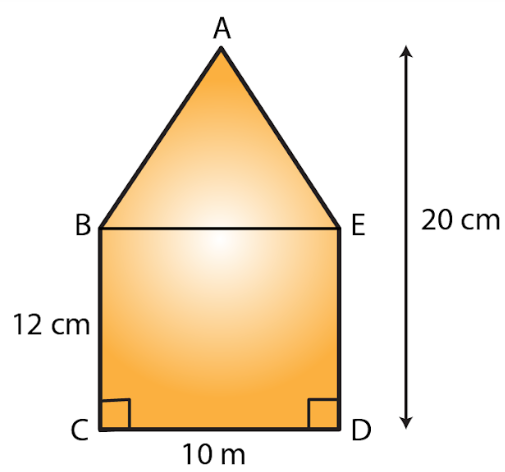Join BE so that we can get rectangle and triangle.

We know that area of rectangle = length x breadth

Area of the rectangle BCDE = CD x DE

= 10 cm x 12 cm

= 120 c m2

Area of triangle = ½ x base x height

Area of Triangle ABE = 1/2 (BE x height of the triangle)

= ½ (10 cm x (20 – 12) cm)

= ½ (10 cm x 8 cm)

= 40 cm2

Hence, Area of the pentagon ABCDE = area of rectangle + area of triangle

= (120 + 40) cm2

= 160 cm2

16. The base of a triangular field is three times its altitude. If the cost of cultivating the field at Rs 24.60 per hectare is Rs 332.10, find its base and height.

Solution:

Let altitude of the triangular field be h m

Then base of the triangular field is 3h m.

We know that area of triangle = ½ x b x h

Area of the triangular field = ½ (h x 3h) = 3h2/2 m2 ……. (i)

The rate of cultivating the field is Rs 24.60 per hectare.

Therefore,

Area of the triangular field = 332.10 /24.60

= 13.5 hectare

= 135000 m2 [Since 1 hectare = 10000 m2] …… (ii)

From equation (i) and (ii) we have,

3h2/2 = 135000 m2

3h2 = 135000 x 2 = 270000 m2

h2 = 270000/3

= 90000 m2

= (300)2

h = 300 m

Hence, Height of the triangular field = 300 m and

Base of the triangular field = 3 x 300 m = 900 m

17. A wall is 4.5 m long and 3 m high. It has two equal windows, each having form and dimensions as shown in Fig. 37. Find the cost of painting the wall (leaving windows) at the rate of Rs 15 per m2.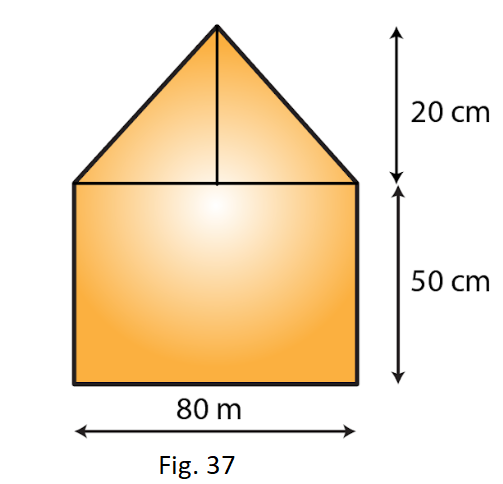Solution:

Given length of a wall = 4.5 m

Breadth of the wall = 3 m

We know that the area of triangle = length x Breadth

Area of the wall = Length x Breadth

= 4.5 m x 3 m = 13.5 m2

From the figure we observed that,

Area of the window = Area of the rectangle + Area of the triangle

= (0.8 m x 0.5 m) + (12 x 0.8 m x 0.2 m) [Since 1 m = 100 cm]

= 0.4 m2 + 0.08 m2

= 0.48 m2

Area of two windows = 2 x 0.48 = 0.96 m2

Area of the remaining wall (leaving windows) = (13.5 – 0.96) m2

= 12.54 m2

Cost of painting the wall per m2 = Rs. 15

Hence, the cost of painting on the wall = Rs. (15 x 12.54)

= Rs. 188.1